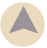# Percentage Change of Value

Calculate the percentage change of a number based on the value's increase or decrease. For example, if your rent or salary increases, easily calculate the percent increase; Or, if the temperature or time of a kiln changes from a previous run, calculate the percent change. Enter the inital value and the new value, and the calculator determines if an increase or decrease occurred and the percent change.

## Percent Change Between Two Number Values

From
To

Examples: From 200 To 400 (Increase); From 400 to 200 (Decrease)

### More Percentage Calculators